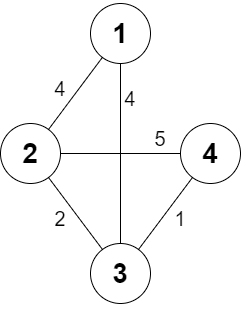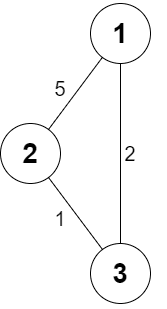##### Welcome to Subscribe On Youtube

Formatted question description: https://leetcode.ca/all/2473.html

# 2473. Minimum Cost to Buy Apples

## Description

You are given a positive integer n representing n cities numbered from 1 to n. You are also given a 2D array roads, where roads[i] = [ai, bi, costi] indicates that there is a bidirectional road between cities ai and bi with a cost of traveling equal to costi.

You can buy apples in any city you want, but some cities have different costs to buy apples. You are given the array appleCost where appleCost[i] is the cost of buying one apple from city i.

You start at some city, traverse through various roads, and eventually buy exactly one apple from any city. After you buy that apple, you have to return back to the city you started at, but now the cost of all the roads will be multiplied by a given factor k.

Given the integer k, return an array answer of size n where answer[i] is the minimum total cost to buy an apple if you start at city i.

Example 1:Input: n = 4, roads = [[1,2,4],[2,3,2],[2,4,5],[3,4,1],[1,3,4]], appleCost = [56,42,102,301], k = 2
Output: [54,42,48,51]
Explanation: The minimum cost for each starting city is the following:
- Starting at city 1: You take the path 1 -> 2, buy an apple at city 2, and finally take the path 2 -> 1. The total cost is 4 + 42 + 4 * 2 = 54.
- Starting at city 2: You directly buy an apple at city 2. The total cost is 42.
- Starting at city 3: You take the path 3 -> 2, buy an apple at city 2, and finally take the path 2 -> 3. The total cost is 2 + 42 + 2 * 2 = 48.
- Starting at city 4: You take the path 4 -> 3 -> 2 then you buy at city 2, and finally take the path 2 -> 3 -> 4. The total cost is 1 + 2 + 42 + 1 * 2 + 2 * 2 = 51.


Example 2:Input: n = 3, roads = [[1,2,5],[2,3,1],[3,1,2]], appleCost = [2,3,1], k = 3
Output: [2,3,1]
Explanation: It is always optimal to buy the apple in the starting city.


Constraints:

• 2 <= n <= 1000
• 1 <= roads.length <= 1000
• 1 <= ai, bi <= n
• ai != bi
• 1 <= costi <= 105
• appleCost.length == n
• 1 <= appleCost[i] <= 105
• 1 <= k <= 100
• There are no repeated edges.